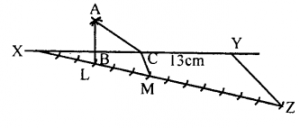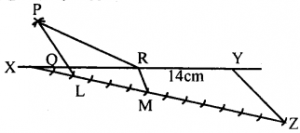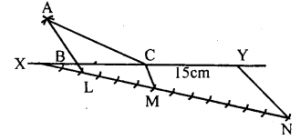# KSEEB Solutions for Class 8 Maths Chapter 12 Construction of Triangles Ex 12.7

In this chapter, we provide KSEEB SSLC Class 8 Maths Chapter 12 Construction of Triangles Ex 12.7 for English medium students, Which will very helpful for every student in their exams. Students can download the latest KSEEB SSLC Class 8 Maths Chapter 12 Construction of Triangles Ex 12.7 pdf, free KSEEB SSLC Class 8 Maths Chapter 12 Construction of Triangles Ex 12.7 pdf download. Now you will get step by step solution to each question.

### Karnataka State Syllabus Class 8 Maths Chapter 12 Construction of Triangles Ex 12.7

Question 1.
Construct a triangle ABC whose perimeter is 13cm and whose sides are in the ratio 3 : 4 : 5.
3 + 4 + 5 = 12Question 2.
Construct a triangle PQR whose perimeter is 14 cm and whose sides are in the ratio 2 : 4 : 5.
2 + 4 + 5 = 11 perimeter is 15cm whose sides are in the ratio 2 : 3 : 4Question 3.
Construct a triangle MNP, whose perimeter is 15 cm and whose sides are in the ratio 2 : 3 : 4.
2 + 3 + 4 = 9All Chapter KSEEB Solutions For Class 8 maths

—————————————————————————–

All Subject KSEEB Solutions For Class 8

*************************************************

I think you got complete solutions for this chapter. If You have any queries regarding this chapter, please comment on the below section our subject teacher will answer you. We tried our best to give complete solutions so you got good marks in your exam.

If these solutions have helped you, you can also share kseebsolutionsfor.com to your friends.

Best of Luck!!